# 4 Math Topics Your Student Needs to Practice More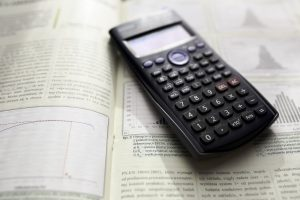Mathematics is a subject that aligns well with the old saying “practice makes perfect.” It is different from other subjects in a sense because it requires patience, time, effort, and (you guessed it) practice.  Some topics in math are generally quicker for students to grasp or are covered rigorously, but there are several math topics your student needs to practice more.  Students need more practice in the topics discussed below for the following reasons:

• The topic is challenging.
• The topic is used often.
• The topic is used on standardized testing (ACT/SAT).

# 4 Math Topics Your Student Need to Practice

## Algebraic Expressions

What: An algebraic expression is an expression composed of constants (numbers), variables (letters), and mathematical operations (+, -, etc.).

Why: Students need more practice with algebraic expressions because…

• Algebraic expressions are a fundamental concept of algebra that continues to get more and more challenging.
• There are many properties and rules involved in solving expressions (order of operations, combining like terms, etc.).
• Expressions are used in more advanced mathematical topics such as calculus and statistics
• Expressions appear on standardized tests.

Example: Simplify the following expression

2(x + 3y) − 4(x − y)

## Exponents

What: An exponent is the number of times a base number is multiplied by itself.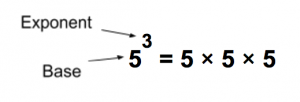Why: Students need more practice with exponents because…

• There are many properties involved with exponents.
• Exponents are seen in other mathematical topics (i.e. solving algebraic expressions).
• Exponents appear on standardized tests.

Example: Simplify the following expression: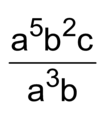## Area and Volume

What: The number of unit squares contained in a two dimensional figure is the area. Volume is the amount of space contained in a three dimensional object.

Why: Students need more practice with area and volume because…

• Understanding area and volume is essential in the real world.
• There are many formulas to find the area and volume of various shapes.
• Area and volume appear in mathematical curriculum every year from about 5th grade on (each year adding more challenging questions). Practicing, especially early on, will give students a great understanding moving forward each year.
• Area and volume are sure to be seen on standardized tests.

Example: Find the area of the figure.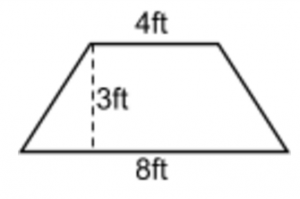## Probability

What: Probability is the likelihood that something is going to happen.

Why: Students need more practice with probability because…

• Probability and statistics are a separate branch of mathematics. Students who continue on in math will need a solid foundation of probability to be successful.
• Probability and statistics are used in every industry of our world today. It is an essential skill for students to learn.
• Probability has an extremely high likelihood of appearing on a standardized test.

Example: In a standard deck of 52 cards, what is the probability of choosing two aces in a row without replacement?

These are four math topics students need to practice more. They are challenging topics that are often used throughout a student’s mathematics education.  Listed below are the solutions to the above examples to see if you may need a little practice on these topics as well! What are you waiting for? Get your students practicing right away!

Author: Nate Baltzer, Writer and Teacher at A Grade Ahead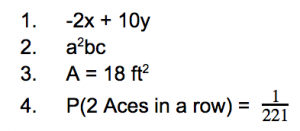## Subscribe

Don't miss the latest article! Stay up-to-date on our blog posts by subscribing below.